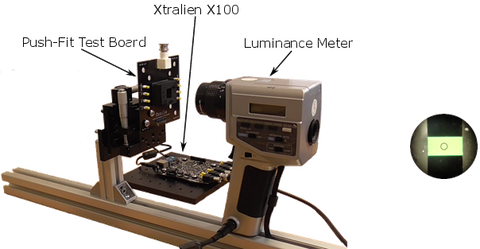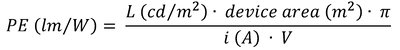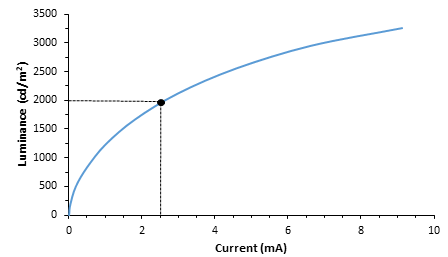FREE shipping to on qualifying orders when you spend or more. All prices ex. VAT. Qualifying orders ship free worldwide! Fast, secure, and backed by the Ossila guarantee. Orders to the EU are processed by our EU subsidiary.

# OLED Testing Guide

This guide gives you an overview of what to consider when characterising an OLED, as well as tips for their measurement.

## OLED Performance Testing

### Parameters

• The typical working range of an OLED (with a pixel size of 5 mm2 or less) is less than 100 mA
• The maximum efficiency of an OLED (see equations below) is typically observed in the brightness range of tens to hundreds of candelas per meter squared (cd/m2).

### Equipment

To characterise our OLEDs, we use our Ossila Source Measure Unit and an Ossila Push-Fit Test Board.

*Please note that the Source Measure Unit used in this guide is an older, discontinued model (X100) that we no longer sell. It has now been replaced with an upgraded version (X200) that has the exact same functionality as the X100, but with added improvements.

As a standard, we set the Source Measure Unit to Range 2, which allows measurements between ±10 mA with a 10 μA resolution, because the pixel size we use (4.5 or 4.0 mm2) leads to currents lower than 100 mA.

The OLED device is loaded into the setup (see figure below) that is composed of the Push-Fit Test Board connected to the source measure unit, and a (third-party) luminance meter that is focused onto one of the pixels to be measured.OLED testing setup (left). Luminance meter is focused and centered onto the pixel (right).

### Data Collection

To acquire the data, we use a Python script (written using Xtralien Scientific Python) that sweeps the pixels from 0 to 10 V and records the luminance.

1. The Source Measure Unit applies the voltage and measures the current within the pixel
2. The luminance meter collects the light emitted from the pixel in cd/m2
3. The script calculates the efficiencies and finds the turn ON voltage
4. The raw collected data of voltage, current and brightness are saved in a '.csv' file

### OLED Characterisation

From the three measured parameters, it is possible to calculate the following quantities:

Current density (j):

Current efficiency (CE):

Power efficiency (PE)*:*The power efficiency calculation is valid within the assumption that the emission of the OLED is isotropic.

For the proper measurement of the External Quantum Efficiency (EQE), an integrating sphere is required, which is beyond the scope of this guide.

### Equipment

What is needed to carry out lifetime experiments is:

1. A source measure unit (source meter) to drive the OLED
2. A probe to measure the intensity of the light emitted over time.

The difference between standard OLED performance testing and OLED lifetime testing is that the probe for lifetime measurements does not need to be calibrated (like the one used for performance testing). This means that the probe for lifetime testing can be something as simple as a photodiode, since what we are interested in is the relative decay of the brightness from an initial value that we set (L0).

Following these guidelines, we developed our OLED Lifetime System (see figure below). The system comprises an Ossila Source Measure Unit, a Multiplexor Test Board, and a photodiode lid.

### Parameters

Lifetime testing typically comes after having tested the OLED's performance (see previous section - OLED performance testing). This is because it is necessary to know what the driving current value (iOLED) is that corresponds to the L0 value (at which it is desired to carry out the experiment).

This information can be easily found from the Luminance vs. Current plot of the OLED (see the figure below).Graphical representation of how to find the current value that corresponds to L0. In this example L0 = 2000 cd/m2 and the correlated value of the current is iOLED = 2.5 mA.

Once you have decided on the L0 value and found the corresponding iOLED value, the experiment can be set up.

• The chosen iOLED current must be kept constant throughout the whole experiment, continuously adjusting the voltage.
• The photocurrent of a photodiode (iPD) is used to follow the luminance decay.

### Procedure

• One source-measure unit (SMU) of the X100 is connected to the OLED to drive it at constant current
• The current range is set to Range 1 (100 mA current limit, 100 μA resolution)
• If the driving current of the OLED is particularly low, Range 2 can also be used (10 mA current limit, 10 μA resolution) in order to have an higher resolution over the control of the current.
• The second SMU of the X100 is connected to the photodiode (PD)
• This current range is set to Range 5 (10 μA current limit, 10 nA resolution).

The role of the multiplexor board is to automatically switch between the pixels on the device and test them all one at a time, setting different iOLED values for each pixel (as necessary).

This is a particularly useful feature for lifetime testing that can optimise the testing time. However, a manual system can also be used, and the OLED Lifetime System is also sold with a manual variant.

The typical shape of the Luminance decay of an OLED, driven at constant current, is shown  in the figure below.

### Data Extrapolation

If the tested device shows a particularly high stability, different strategies can be applied to reduce the testing time.

In reality, a hypothetical lifetime of 10,000 hours would require an experiment longer than 1 year. For this reason, it is vital to have the means to run accelerated tests and extrapolate the lifetime at lower operational conditions.

There are different ways of doing so, and we will elaborate on these two methods of extrapolation here.

#### 1) Stretched Exponential Decay (SED)

The first method involves taking a portion of the initial data, and then using a model to fit the data to extrapolate the test results (had the experiment continued to run). One of the most-used fit models is the Stretched Exponential Decay (SED) :

Where L(t) is the value of the luminance over time, L0 the initial value of the luminance, t is the time, β and τ are fitting parameters.

Even though this is a powerful tool for lifetime testing, the accuracy of the extrapolation depends heavily on the amount of actual data acquired initially.

The second method is to run highly-accelerated lifetime tests at different initial L0 values, and use the obtained lifetime data to extrapolate what the results for lower L0 values would have been.

Let’s put this into context. In 1996, Van Slyke et al  described the OLED degradation process as “coulombic”, i.e. determined only by the total charge injected into the device. Following this assumption, in 2002, Popovic and Aziz  demonstrated that the product between the initial luminance (L0) of the lifetime test and the lifetime obtained (LT50 if considering 50% decay from L0) obtained is a constant.

Deviations from the equation above can be taken in account introducing an acceleration factor n.

This factor can easily be determined experimentally by running lifetime tests for different L0 values.

The latest equation can be rewritten in the form:

By plotting the logarithm of lifetime values obtained against the initial luminance values, n can be extrapolated as the slope of the linear fit, as shown in the graphical example below.

From this plot, it is therefore possible to extract information of lifetimes for lower L0 values.

### References

 C. Kristukat, T. Gerloff, T. Hoffmann, and K. Diekmann, determination procedure for OLED,” in Organic light-emitting diodes, 1st ed., Alastair Buckley, Ed. Cambridge: Woodhead Publishing, 2013, pp. 601–634.

 S. a. Van Slyke, C. H. Chen, and C. W. Tang, Appl. Phys. Lett., vol. 69, no. 15, p. 2160, 1996.

 H. Aziz, Z. D. Popovic, and N. Hu, Appl. Phys. Lett., vol. 81, no. 2, pp. 370–372, 2002.This project has received funding from the European Union’s Horizon 2020 Research and Innovation Programme under grant agreement No 674990.

### Contributing Authors

Written by

Marco Colella

PhD Student Collaborator